# More Problem Solving Power: Exploiting Prediction Models and Statistical Software in a One-Semester Course

Joe H. Ward, Jr.
University of Texas at San Antonio

Robert L. Fountain
Portland State University

Journal of Statistics Education v.4, n.3 (1996)

Copyright (c) 1996 by Joe H. Ward, Jr., and Robert L. Fountain, all rights reserved. This text may be freely shared among individuals, but it may not be republished in any medium without express written consent from the authors and advance notification of the editor.

Key Words: Linear models; Undergraduate teaching techniques.

## Abstract

Many widely-adopted college textbooks that are designed for a student's first (and possibly last) statistics course have incorporated new trends in statistical education, but are organized in a manner that is still driven by a traditional computational, rather than a conceptual, framework. An alternative approach allows for the treatment of many seemingly-unrelated conventional procedures such as one- and two-sample t-tests and analyses of variance and covariance under a unifying prediction model approach. Furthermore, this approach, combined with the power of modern statistical software packages, prepares the student to solve problems beyond the scope of traditional procedures. Students will appreciate the acquisition of practical research capabilities and might even be stimulated to continue their study of statistics.

# 1. Introduction

1 A traditional one-semester introductory statistics course includes numerous topics, each given a very brief treatment. These topics typically include numerical and graphical data analysis tools, introductions to combinatorics, set theory, and probability, applications of several discrete and continuous probability distributions, sampling distributions of the sample mean and standard deviation, confidence intervals and hypothesis tests for means, proportions, and variances of one and two populations, simple linear regression, analysis of variance, and $\chi^2$ tests of independence. This approach in many instances leaves the students with the inability to attack common problems, having spent so little time with each technique. It also fails to demonstrate to the students that there are many more problems that could be solved using techniques very closely related to those which they have learned; the traditional approach, instead, encourages students to pigeonhole new problems into a few narrow categories.

2 Many widely-adopted statistics texts have incorporated new trends in introductory statistics education but still contain traditional restrictions. For example, some statistic texts require a two-way ANOVA to have equal sample sizes in each cell. This restriction prohibits the student from working with the more practical situations when the cell frequencies are unequal or when the cell category has no observations. Discussions of the confusing results obtained in missing cell'' situations can be found in Freund (1980) and Jennings and Ward (1982).

3 This preservation of the traditional (and restrictive) algorithms deprives the student of the opportunity to explore the power of computing-intensive data-analysis models. It is suggested below that a prediction model approach, aided by the widespread availability of computers, can be introduced to the beginning statistics students, empowering them to expand their analysis capabilities to more advanced problems. There have been many stimulating and valuable writings and presentations concerned with improving teaching the introductory statistics course. These emphasize using real data to teach statistical concepts rather than techniques, and suggest keeping the amount of probability to a minimum. Bentley, Cobb, Lock, Moore, Parker, and Witmer (1994) have assembled a valuable collection of student activities and projects designed to encourage educators to expand their concepts of teaching statistics beyond lectures and doing traditional homework problems. Witmer (1992) has designed a textbook supplement to present the tools of analysis in an engaging manner and at a level that is accessible to students in elementary classes. Moore and McCabe (1993) present a refreshing approach by beginning their Introduction to the Practice of Statistics by looking at data distributions and relationships and producing data before discussing formal ideas of probability.

4 Certainly the many valuable activities that have been developed to improve students' understanding and interest in data analysis should be incorporated into an introductory course. However, the introduction of digital computers has long-ago opened the opportunity for beginning students to exploit the analysis power of more computing-intensive procedures.

5 The method suggested here provides beginning students with increased data analysis capability by combining a prediction model approach with high-speed computing power. It possesses several important advantages over traditional methods:

• Many of the seemingly unrelated traditional procedures can be accomplished under a single framework.
• Since what they learn is generalizable to many future situations, students will be able to create new models for unique problems.
• Students will be able to solve more problems with less computational burden, since the use of statistical software packages allows for solutions to complex prediction problems.

6 The approach is based on earlier publications by Bottenberg and Ward (1963), Ward (1969), Ward and Jennings (1973), and Ward (1991). It has been discussed previously by Fountain and Ward (1993). Experiences with the prediction model approach are presented in Section 5 and some suggestions for implementing the approach in the introductory course are presented in Section 6.

# 2. The Prediction Model Approach

7 The prediction model method has two aspects. First, it synthesizes many standard procedures within the single framework of the linear model. Second, a problem-solving system is used, in which the students learn to adapt the model to fit the needs of each individual problem with which they are faced. The goals of this approach are:

• The students will have the power to solve some meaningful problems and to recognize other problems well enough to request interesting analyses from statisticians.
• The students will be able to identify research problems and formulate statistical models with which to analyze them.
• The students will develop the ability to distinguish between the procedures available in common statistical software packages.
• The students will be capable of interpreting the output obtained from such software and of preparing the results for presentation in technical reports.

8 In traditional one-semester statistics courses, so little time usually remains when regression and analysis of variance are presented that these topics are given extremely brief treatments or are omitted entirely. The prediction model approach integrates these topics with one- and two-sample t-tests and allows for expansion to more complex models. Using the same general form of the prediction model and adapting it to meet the needs of each problem, estimation and hypothesis testing may be done for each of the following:

1. The value of a single population mean (one-sample t-test).
2. The relationship between two population means (two-sample t-test).
3. The relationships among means of several populations (one-way ANOVA).
4. The relationships among means of several populations, controlling for a blocking attribute (randomized complete block design).
5. The comparison of cell means, allowing for the interaction between two predictor attributes (two-way ANOVA with interaction).
6. The effect of a single continuous predictor attribute (simple linear regression).
7. The effects of several continuous predictor attributes (multiple regression).
8. The effect of a continuous predictor attribute together with a categorical predictor attribute (analysis of covariance, or ANOCOVA).

# 3. The Unifying Model

9 In this section, we will give precise formulations of the models used in eight types of analyses, showing how they all fit into the unified prediction model approach. The use of Greek letters and subscripts would not be necessary when introducing the concept of prediction models to inexperienced students. Section 6 contains some strategies for the classroom presentation of the material.

10 The general form of the prediction model common to all of the problems listed above is, for i = 1, ..., n: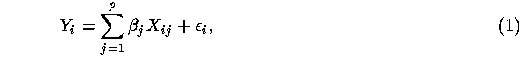where Y represents the response being measured, X_1, X_2, ..., X_p are the predictor attributes, $\epsilon$ is an error variable with zero mean and constant variance, and n is the number of observations. For a continuous predictor, X_ij is the value of the jth predictor at the ith observation. For a categorical predictor with k mutually exclusive categories, the model would contain k indicator variables, each taking on the value 1 when the observation falls in that category and 0 otherwise. To include a constant in the model, a predictor is defined that takes on the value 1 for all observations. Even at the introductory level, it can be explained that the coefficients in the prediction equation are chosen so that the differences between the actual and predicted responses are as small as possible. The concept of an error sum of squares as a numerical measure of the total difference is not a difficult one to convey at this point.

11 The students learn to translate stated problems into appropriate models, formulating suitable hypotheses. Some discussion of the general ideas behind hypothesis testing must be introduced, including the interpretation of the conclusions, reject H_0'' and fail to reject H_0''. The restricted and full models are created to correspond to the null and alternative hypotheses, respectively. Until the point in the course where probability distributions are formally presented, issues concerning violations of the error assumptions can be postponed, and the F statistic can be introduced and interpreted merely as a quantifier of the difference between the full and reduced model error sums of squares:Variations on the model

12 The versions of the full and restricted models needed to carry out the eight analyses mentioned earlier are shown below. In some cases, several different hypotheses may be tested, and representative examples have been selected. Throughout the following, U_i = 1 for i = 1, 2, ..., n.

1. One-sample t-test. The full model is: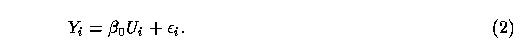To test the null hypothesis that the mean of the population equals a specified value $\mu_0$, the restricted model is:2. Two-sample t-test. The full model is: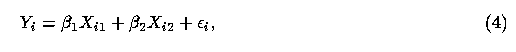where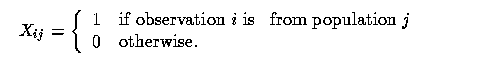Notice that this is a cell means'' model. That is, the least squares estimates of $\beta_1$ and $\beta_2$ will be the sample means of the dependent variable Y for samples 1 and 2.

To test the null hypothesis that the means of the two populations are equal, the restricted model is: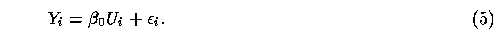The least squares estimate of $\beta_0$ will be the overall sample mean of Y.

3. One-way ANOVA. The full model is: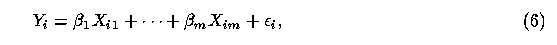where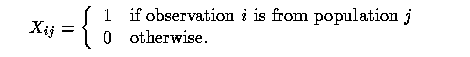This is another cell means model, since no grand mean term appears on the right hand side. The estimates of the $\beta_i$s will again be the sample means of Y for samples 1 through m.

To test the null hypothesis that the means of the populations are equal, the restricted model is:4. Complete block design. The full model is:whereNote that the blocking index k runs from 1 to r-1 if there are r blocks. This prevents overparameterization, a situation in which the parameter estimates are not unique. To test the null hypothesis that there is no treatment effect, the restricted model is: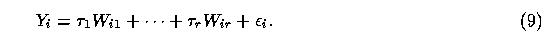5. Two-way ANOVA. The full model is: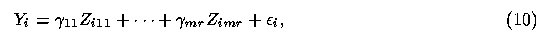whereNote that, in this parameterization, there are no main effect'' and interaction'' terms. Instead, the parameters $\gamma_{11}$, ..., $\gamma_{mr}$ are the means of the cells representing specific combinations of the treatments. To test the null hypothesis that there is no interaction between treatments, the restricted model is:Note that (11) is the same as (8). To test the null hypothesis that there is no main effect for the second treatment, given that there is no interaction, the full model is the previous restricted model (11), and the new restricted model is:6. Linear regression. The full model is:To test the null hypothesis that the slope is zero, the restricted model is: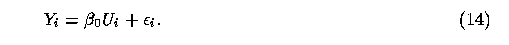7. Multiple regression. The full model is: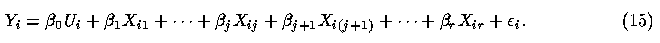To test the null hypothesis that the coefficients $\beta_{j+1}$, ..., $\beta_r$ equal zero, the restricted model is:8. ANOCOVA. The full model is: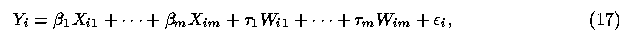where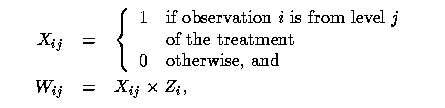where Z is a quantitative predictor. To test the null hypothesis that the slopes are the same for each level of the treatment, the restricted model is:To test the null hypothesis that there is no treatment effect, given that the slopes are equal, the full model is the previous restricted model (18), and the new restricted model is: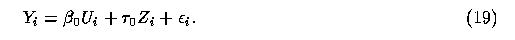Other models

13 The eight models presented above represent the most common problems presented in traditional introductory statistics courses. Some of these, such as the randomized complete block design, two-way analysis of variance, multiple regression, and the analysis of covariance, are usually covered in a second-semester statistics course. The prediction model approach allows these topics to be presented at the same time as simple linear regression and one-way analysis of variance. Other subjects such as polynomial regression and linear and polynomial spline models may also be covered using the same method. Even in the context of standard models, students can be encouraged to form conjectures beyond the usual hypotheses that certain coefficients are equal to each other or are equal to zero. For example, within the one-way ANOVA framework, it is possible to test for constant differences between the means at successive levels of the treatment, as is illustrated in the next section. The students, as a result, may grasp the similarities between ANOVA and linear regression. In addition, the missing cells problem is alleviated using the method described. If there are one or more missing cells in a two-way ANOVA (see variation 5 above), then the full model (10) will contain only those binary predictors (and corresponding parameters) associated with cells containing observations. Then the student can state hypotheses of interest in terms of the full model. This assures that the results obtained from the regression model analysis will reflect the hypothesis of interest. And, of most importance, it protects the student from the risk of unknowingly using output of packaged ANOVA procedures that may give results that answer uninteresting questions. This has been discussed by Freund (1980) and Jennings and Ward (1982). Furthermore, this approach allows the student to clearly communicate the hypotheses being tested, so that a reader of the research report knows exactly what is being done. For example, some packaged ANOVA programs may label as a main effects'' test one that assumes equally-weighted cell means, while others may label as a main effects'' test one that uses weights based upon cell frequencies. This has been discussed by Jennings and Green (1984).

# 4. Example

14 The following example uses a data set that would be very difficult to analyze using only the methodology presented in a typical introductory statistics course. The purpose of its inclusion here is threefold:

1. To demonstrate the breadth of questions that can be addressed by using the general prediction model approach,
2. To illustrate the shortcomings of the traditional approach, and
3. To show how statistical software can be used to fit the full and reduced models.

15 We will continue the formal presentation in this section, in order to precisely state the hypotheses being tested in response to each of the questions posed. The next two sections contain a more informal approach, with suggested methods of presentation in the classroom. The example uses PROC REG in Version 6 of SAS (1989) to analyze a data set that appears in Glass and Stanley (1970, p. 112) and is shown in Table 1. There are eight equally-spaced age groups, and the measure of performance (Y) is the digit-symbol subtest of the Wechsler Adult Intelligence Scale. The mean scores for each age are plotted in Figure 1. A student in a typical introductory statistics course would have only a one-way ANOVA and a simple linear regression analysis as tools with which to analyze the data. As can be seen from the figure, neither of these would capture the more interesting aspects of the data. We wish to investigate three questions:

1. Does the mean value of Y differ among the eight age groups? In terms of expected values we can express the hypothesis aswhich can easily be expressed in the SAS TEST statement as shown below.

2. Is there a constant difference (linear trend) in means? In terms of expected values we can express the hypothesis asThese restrictions can easily be imposed with the SAS TEST statement.

3. Is there a constant second-order difference (quadratic trend) in means? In terms of expected values we can express the hypothesis aswhich can be simplified for ease of expression in the SAS statement as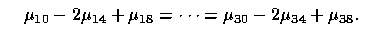16 The MODEL statement in PROC REG is used to describe the full model, and the TEST statement is used to specify the restrictions. Figure 2 (see Appendix) shows the SAS program that generates the required analyses.

         Table 1:  Digit-symbol subtest scores in 8 age groups

-------------------------------------------
Age to nearest year
-------------------------------------------
10   14    18    22    26   30   34   38
-------------------------------------------
7    8     9    11     9    8    7    8
8    9    10    11    10    9    9
9   10    11    12    11    9   10
9   11    12    12         10
10
-------------------------------------------
Means
8.6  9.5  10.5  11.5  10.0  9.0  8.7  8.0
-------------------------------------------


17 The output of the SAS program is shown in Figure 3 (see Appendix). At the 5% level of significance, the hypothesis of no differences in mean response for the eight age groups would be rejected. The hypothesis of constant differences would be rejected. The hypothesis of constant second-order differences would fail to be rejected. Thus, it appears that there may be a quadratic trend in the mean value of Y across the eight age groups. Using the traditional approach to analysis of variance in introductory courses, with its emphasis on testing for main effects, the preceding analysis would be well beyond the capabilities of the vast majority of students.

18 In variations 5 and 8, discussed in the previous section, several conditional hypothesis tests were mentioned. These may easily be performed in PROC REG by placing constraints on the full model with the RESTRICT statement.

19 Note: While the example shown above used the SAS statistical software, any appropriate software package can be used to carry out the computational requirements for the analyses.

# 5. Experiences with the Prediction Model Approach

20 There has been a wide range of experiences with various approaches to implementation of the more formal presentation above (THE UNIFYING MODEL).

21 The earliest experiences with the approach began in the 1950s, just as expensive, slow-speed computers became available. The first target group consisted of mostly research psychologists at the Air Forces Personnel Research Laboratory at Lackland Air Force Base. This group had been trained with pre-computer methods of data analysis. Also, at the same time, the approach was presented to a small group of high school students. One of the high school students received recognition from the Westinghouse Talent Search for his paper titled A Vector Approach to Statistics''. These experiences formed the basis for Applied Multiple Linear Regression by Bottenberg and Ward (1963).

22 In 1964 the National Science Foundation sponsored a short course in the use of regression analysis and computers for behavioral scientists at the University of Texas at Austin. This was followed for several years by Presessions at the annual meetings of the American Educational Research Association (AERA). The AERA Special Interest Group (SIG) in Multiple Linear Regression was formed by alumni of these Presessions, and the SIG now has additional members.

23 The first author has conducted short courses using this approach and has included it as part of non-calculus based introductory statistics courses at St. Mary's University of San Antonio, The University of Hawaii, The University of Texas at San Antonio, and Clemson University.

24 In recent years, focus has been directed toward high school students. Since 1991 the first author has tried various strategies for introducing the approach to high school students in grades 9 - 12. Some of these students have received special recognition for their use of statistics in Regional and International science fair projects. In the 1996 American Statistical Association Project Competition, an entry based on this approach received a special prize award to the team submitting the statistics project making the best use of a computer''.

25 There have been no formal assessment experiments for either college or high school students to estimate the value of this approach for introductory statistics students. While it would be of interest to compare instruction using the prediction model approach with more traditional courses, it may be difficult. As in many such studies comparing different approaches, it is necessary to evaluate outcomes for learning objectives common to both methods. The prediction model approach, although related to regression and linear models, is usually not among the learning objectives for an introductory statistics course. The approach is suggested as one component of a statistics course which, if properly introduced to each particular audience, should give students the research power not otherwise available.

# 6. Strategies for Implementing the Approach

26 A prediction model approach can be used not only in an introductory college-level course but also for some high school students. Many high school students have had experience with functions without errors,'' and an increasing number of high school students are familiar with functions with errors'' (least-squares fitting) ( Foerster 1986). These concepts lead naturally into the prediction model approach (Ward and Foerster 1991).

27 The approach starts with one or more interesting problems and introduces topics to address the problems only as the topics are needed. This means, of course, that probability ideas are not introduced until much later than in some traditional courses. Some courses still approach the subject with techniques that were appropriate before computers. And when the computer is used it is to process the algorithms of pre-computer days. The use of packaged algorithms can lead to disastrous results ( Freund 1980, Jennings and Ward 1982).

28 On the first day we try to show the students that they will be able to do things with the course objectives that they cannot do without the course. We try to get as quickly as possible to the question, How do we control for the uncontrollable?'' (Ward and Niland 1994).

29 Students move quickly to the natural language discussion of how to predict a dependent (or response) variable from one attribute, then how to control for a second variable that might confound, or contaminate, the results.

30 Problems of interest should be chosen for the particular audience. Examples of four different real-world problem situations that have been used with various audiences are presented below.

1. To win a bet as to which basketball player will score more points in the next game.
2. To predict which business, Sea World of Texas or Sea World of Florida, will earn the most profit next year.
3. To compare the effectiveness of teachers.
4. To compare the sizes of babies born to mothers who smoke and mothers who do not smoke.

Then we can discuss some questions of interest:

1. Is there a difference between the performance of player x and player y?
2. Is there a difference in profits between Sea World of Texas and Sea World of Florida?
3. Is there a difference between student performance measures for various teachers?
4. Is there a difference in birth weights between babies born to mothers who smoke and mothers who do not smoke?

31 From here we brain-storm what might bother you'' about these questions. This leads to making a list of variables that might confuse, confound, contaminate, or mess up'' our investigations. We discuss how nice it would be to control for the uncontrollable. This leads to the idea, If you can't control it, then try to measure' it''. For Example 1 above we might generate a list of variables that we might like to control'' such as:

• Home vs. away games
• Who is guarding the players
• Injury status

For Example 2 above:

• Weather conditions (rainfall, temperature)
• Other events in the area
• Special attractions

For Example 3 above:

• Quality of the students (pretest scores)
• Socio-economic status (free lunch)
• Ethnic category

For Example 4 above:

• Gestation period
• Ethnic background
• Age of mother

32 After introducing two predictor attributes (or factors) into our models, it is important to investigate the presence or absence of interaction between the two attributes. Detailed discussions are introduced regarding the various conclusions that can be made based upon the analysis of possible interaction. For Example 3 above, if it is found that there is a strong interaction between Teachers and Pretest Scores, then better student performance might be obtained by assigning a particular teacher to a specific student. And, if it is concluded that there is no interaction, then it may be appropriate to assign any student to any teacher.

33 We indicate to the statistics student that there are some powerful things to be accomplished by combining a prediction model approach with the computer to answer questions of interest.

34 The data analysis and problem solving strategies are based on four major ideas:

• Prediction
• Uncertainty
• Modeling
• Optimization
• With these four ideas we can systematically investigate interesting real-world problems.

35 The students discuss various ways of making predictions of variables of interest. This leads to the use of means of subsets of data for making predictions. Most students at all levels have experienced the use of averages as indicators for evaluation or assessment. The first prediction models that are used involve cell-means models. As mentioned earlier, the least-squares solutions to these models produce averages of the dependent variable as the predicted values. The fact that the computer solution produces results (cell means) that the students need to investigate their questions of interest builds confidence in the use of prediction models and least squares estimates.

36 The discussion includes reasons for uncertainty in the accuracy of predictions: measurement errors, unknown information that might improve prediction, sampling errors, and inadequate ways of combining the predictor information.

37 This leads to a more formal idea of a model to represent the relationship between the variable to be predicted (dependent variable) and the relevant predictor information. Examples of such representations are:

• Dependent Variable = Function of Predictor Information + Error
• Data = Fit + Residual
• Data = Model + Error
• Y = Prediction + Error
• Y = P + E

38 After reasonable prediction models are developed it is important to use those predictions to make practical decisions that optimize some value indicator'' (objective function). Objective functions might involve combinations of several indicators such as cost, satisfaction, profit, or pollution.

39 One of the key components of the approach is to allow students to discuss and arrive at their own indicators'' of outcomes (response) that are to be predicted. Then past experience has shown that students will tend to make predictions of the responses using averages (sample means) of the response values corresponding to members of mutually exclusive groups, such as Male/Female, Tall/Medium/Short, or MethodA/MethodB/MethodC.

40 After students have decided that they can make some useful predictions using averages, which are easy to do without a computer, they can learn to use a computer to perform these simple'' calculations. But, most important, they learn to use the computer to solve computationally intensive models that they have personally created, and for which there may not be canned'' algorithms. Students soon become able to create models to fit the problem'' rather than trying to squeeze the problem into existing models''.

41 For introductory statistics courses there usually are many more objectives than time permits. For example, in some college courses, the analysis of variance and analysis of covariance are made optional if time permits''. These topics may be optional because of the special notation that is required to use the pre-computer algorithms. The student may already have been introduced to simple linear regression, so it seems appropriate to continue with regression to develop models that include the omitted topics. With a prediction model approach, many seemingly-different standard'' models can be accomplished with one general strategy. And, most important, students have the power to create their own models.

42 Consideration must be given to the relationship of the standard algorithms to new approaches. Eventually, some of the more traditional objectives might be replaced by newer, more powerful approaches. Even with the inclusion of a prediction model approach, there is perhaps an obligation by the instructors to provide students with more traditional ideas so that they can communicate with others. With high school students, there is more freedom to introduce this approach since there will be future opportunities for more traditional course content.

43 Providing first-statistics-course students with the combined power of prediction model strategies and computer software will enable them to approach practical problems beyond the scope of some traditional courses. Students will appreciate the acquisition of practical research capabilities and might even be stimulated to continue their study of statistics.

## Acknowledgments

The authors wish to thank Earl Jennings [University of Texas at Austin] and Robert Mason [Southwest Research Institute] for their many helpful comments. They also thank the referees for suggesting many improvements to the manuscript.

# Appendix

      DATA;
LABEL Y = 'DIGIT SYMBOL SUBTEST OF WAIS';
INPUT Y AGE @@;
X10=0;X14=0;X18=0;X22=0;X26=0;X30=0;X34=0;X38=0;
IF AGE EQ 10 THEN X10 =1; ELSE IF AGE EQ 14 THEN X14 =1;
ELSE
IF AGE EQ 18 THEN X18 =1; ELSE IF AGE EQ 22 THEN X22 =1;
ELSE
IF AGE EQ 26 THEN X26 =1; ELSE IF AGE EQ 30 THEN X30 =1;
ELSE
IF AGE EQ 34 THEN X34 =1; ELSE IF AGE EQ 38 THEN X38 =1;
CARDS;
7 10  8 14  9 18 11 22  9 26  8 30  7 34  8 38
8 10  9 14 10 18 11 22 10 26  9 30  9 34
9 10 10 14 11 18 12 22 11 26  9 30 10 34
9 10 11 14 12 18 12 22       10 30
10 10
PROC REG;
TITLE1 'WAIS Example';
TITLE2 'Three Hypothesis Tests';
MODEL Y = X10 X14 X18 X22 X26 X30 X34 X38/NOINT;
NO_DIF:   TEST X10=X14,X14=X18,X18=X22,X22=X26,
X26=X30,X30=X34,X34=X38;
CON_DIF:  TEST X10-X14=X14-X18,X14-X18=X18-X22,
X18-X22=X22-X26,X22-X26=X26-X30,
X26-X30=X30-X34,X30-X34=X34-X38;
X14-2*X18+X22=X18-2*X22+X26,
X18-2*X22+X26=X22-2*X26+X30,
X22-2*X26+X30=X26-2*X30+X34,
X26-2*X30+X34=X30-2*X34+X38;


                                     WAIS Example
Three Hypothesis Tests
Model: MODEL1
NOTE: No intercept in model. R-square is redefined.
Dependent Variable: Y          DIGIT SYMBOL SUBTEST OF WAIS
Analysis of Variance
Sum of         Mean
Source          DF      Squares       Square      F Value       Prob>F
Model            8   2614.13333    326.76667      262.815       0.0001
Error           20     24.86667      1.24333
U Total         28   2639.00000
Root MSE       1.11505     R-square       0.9906
Dep Mean       9.60714     Adj R-sq       0.9868
C.V.          11.60645
Parameter Estimates
Parameter      Standard    T for H0:
Variable  DF      Estimate         Error   Parameter=0    Prob > |T|
X10        1      8.600000    0.49866488        17.246        0.0001
X14        1      9.500000    0.55752429        17.040        0.0001
X18        1     10.500000    0.55752429        18.833        0.0001
X22        1     11.500000    0.55752429        20.627        0.0001
X26        1     10.000000    0.64377360        15.533        0.0001
X30        1      9.000000    0.55752429        16.143        0.0001
X34        1      8.666667    0.64377360        13.462        0.0001
X38        1      8.000000    1.11504858         7.175        0.0001
Dependent Variable: Y
Test: NO_DIF   Numerator:      4.2588  DF:    7   F value:   3.4253
Denominator:  1.243333  DF:   20   Prob>F:    0.0143
Test: CON_DIF  Numerator:      4.9184  DF:    6   F value:   3.9558
Denominator:  1.243333  DF:   20   Prob>F:    0.0090
Test: QUAD_DIF Numerator:      1.3677  DF:    5   F value:   1.1000
Denominator:  1.243333  DF:   20   Prob>F:    0.3912



# References

Bentley, D. L., Cobb, G. W., Lock, R. H., Moore, T. L., Parker, M. R., and Witmer, J. A. (1994), Teaching the Introductory Statistics Course,'' American Statistical Association Short Course, Toronto, Canada.

Bottenberg, R. A., and Ward, J. H., Jr. (1963), Applied Multiple Linear Regression, PRL-TDR-63-6, AD-413 128, Lackland AFB, TX: 6570 Personnel Research Laboratory, Aerospace Medical Division.

Foerster, P. A. (1986), Precalculus with Trigonometry: Functions and Applications, Menlo Park, CA: Addison-Wesley.

Fountain, R. L., and Ward, J. H., Jr. (1993), Regression Models and Software Packages: Synthesizing Traditional Procedures in a One-Semester Statistics Course,'' in 1992 Proceedings of the Section on Statistical Education, American Statistical Association, pp. 375-379.

Freund, R. J. (1980), The Case of the Missing Cell,'' The American Statistician, 34, 94-98.

Glass, G. V., and Stanley, J. C. (1970), Statistical Methods in Education and Psychology, Englewood Cliffs, NJ: Prentice-Hall, Inc.

Jennings, E., and Green, J. L. (1984), Resolving Nonorthogonal ANOVA Disputes Using Cell Means,'' Journal of Experimental Education, 52, 159-162.

Jennings, E., and Ward, J. H., Jr. (1982), Hypothesis Testing in the Case of the Missing Cell,'' The American Statistician, 36, 25-27.

Moore, D. S., and McCabe, G. P. (1993), Introduction to the Practice of Statistics (2nd ed.), New York: W. H. Freeman.

SAS Institute Inc. (1989), SAS/STAT User's Guide, Version 6 (Vol. 2, 4th ed.), pp. 1351-1456.

Ward, J. H., Jr. (1969), Synthesizing Regression Models -- An Aid to Learning Effective Problem Analysis,'' The American Statistician, 23, 14-20.

Ward, J. H., Jr. (1991), Problem Solving Through Data Analysis, Texas Prefreshman Engineering Program (TexPREP), The University of Texas at San Antonio.

Ward, J. H., Jr., and Foerster, P. A. (1991). Integrating Statistics into the Secondary Curriculum,'' in Proceedings of the Third International Conference on Teaching Statistics, ISI Permanent Office, The Netherlands.

Ward, J. H., Jr., and Jennings, E. (1973), Introduction to Linear Models, Englewood Cliffs, NJ: Prentice-Hall, Inc.

Ward, J. H., Jr., and Niland, L. (1994), `Empowering High School Students to Exploit Statistical Models and Software for Research Projects,'' presented at the 1994 Joint Statistical Meetings, Toronto, Canada.

Witmer, J. A. (1992), Data Analysis: An Introduction, Englewood Cliffs, NJ: Prentice-Hall, Inc.

Joe H. Ward, Jr.
San Antonio, TX 78228-2402

joeward@tenet.edu

Robert L. Fountain
Department of Mathematical Sciences
Portland State University
Portland, OR 97207-0751

fountain@alamo.mth.pdx.edu